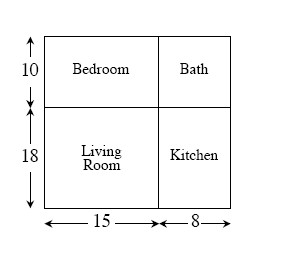### Home > AC > Chapter 1 > Lesson 1.1.4 > Problem1-39

1-39.

Susan's apartment is shown below. Assuming that all rooms are rectangular, find the quantities described below. All measurements are in feet.1. Find the area of her living room.

Area is the total square units of a shape.

Area of a rectangle is base multiplied by height.

Base $=15$ Height $=18$

$15(18)=270$

Reminder! Don't forget your units. $\text{ft} \left(\text{ft} \right) = \text{ft}^2$

$270 \text{ ft}^2$

1. Find the area of her entire apartment.

Follow the steps in part (a).

$644 \text{ ft}^2$

1. How much larger than her bedroom is her living room?

Find both and subtract the smaller area from the larger area.